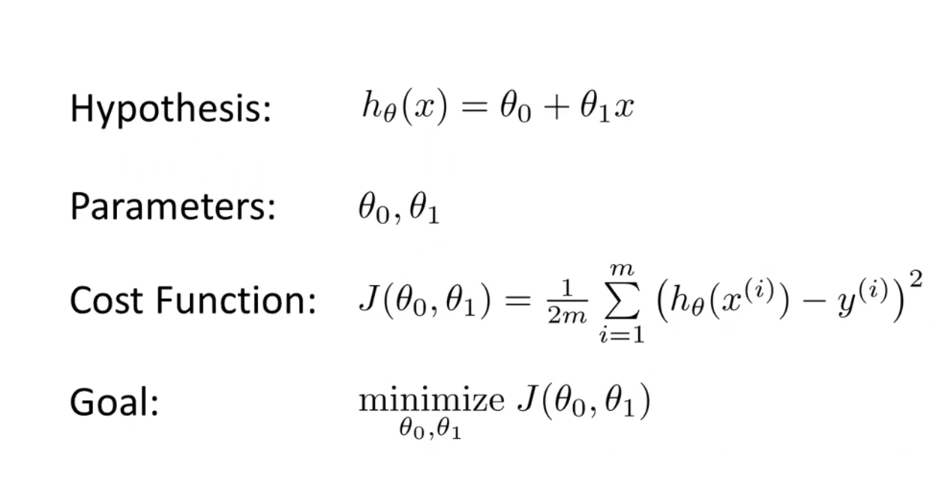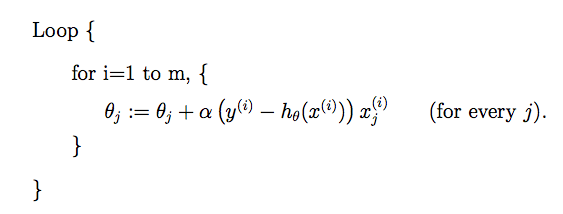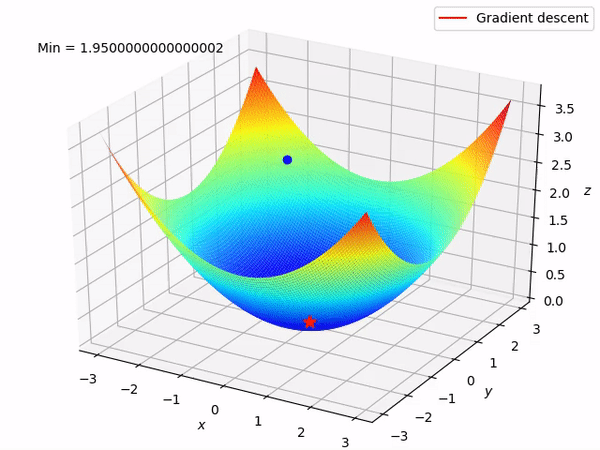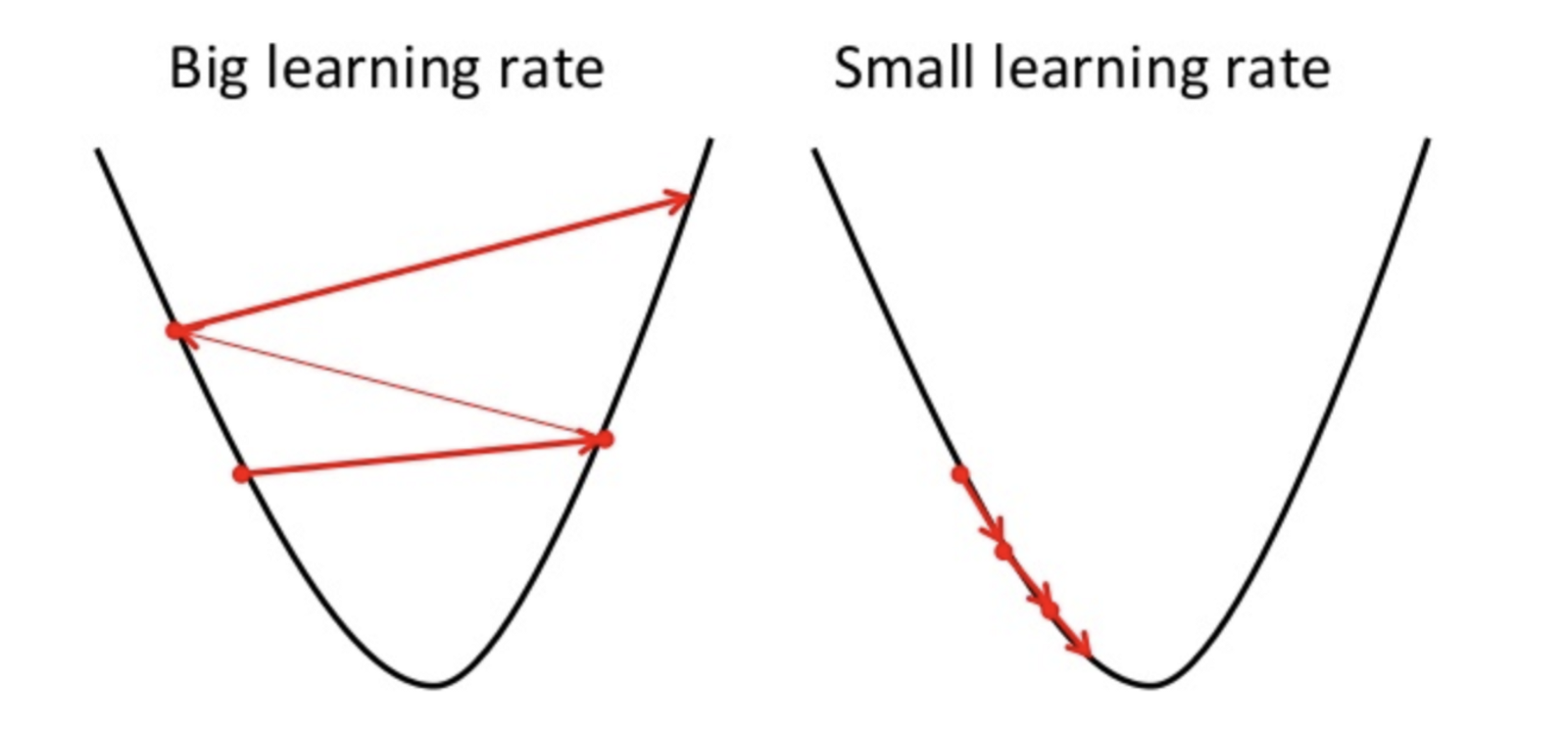×

Search anything:

#### Artificial Intelligence Machine Learning (ML) gradient descentGet this book -> Problems on Array: For Interviews and Competitive Programming

Gradient descent algorithm is one of the most popuarl algorithms for finding optimal parameters for most machine learning models including neural networks.The basic method that this algorithm uses is to find optimal values for the parameters that define your ‘cost function’.Cost function is a way to determine how well the machine learning model has performed given the different values of each parameters.

For example,

the linear regression model, the parameters will be the two coefficients, theta 1 and theta 2.In this scenario, m is the number of points we have in our dataset.

Our goal is to reduce the cost function,for doing that we find the optimal values for theta1 and theta2.(We start with random values for both these parameters)

We use this simple formula to find the optimal values for theta1 and theta2.Here j takes values 0 and 1.
alpha is a parameter known as the leaning rate.
So why does this work?

### What is y(i)-h(x(i))x(j)?

If you are familiar with differentiation, then this is the differential of the cost function with respect to ‘h theta’ multiplied by ‘-1’.

### Why are we differentiating?

To find the slope at that point.
If the slope is positive then the function is increasing at that point,then to reduce the cost ‘h theta’.So we will get a lesser value for cost function when we decrease the value of theta (which is why we multiply by ‘-1’).The vice versa also works.In the above gif,assume the bowel shaped graph is our cost function.The blue point is our starting point and the red point denots he least value that our cost function can take.

From the blue point we try to reduce cost function.At the blue point the graph has a positve slope,so we have to reduce the slope.So we reduce the paramete value and try again.This step is done either for a fixed number of times or unti we reach the minimum value for cost function.

### Now what is alpha?

Alpha also known as learning rate is a parameter that judges how big a jump you should make each step.A bigger learning rate would mean you take a bigger jump in whatever direction (depends on your slope) and vice versa.
The value that you set for alpha depends on the problem and the dataset.

As mentioned in the introduction,this algorithm is probably one of the most used algorithms and is used to optimize parameters and has a big application in the field of deep learning.#### JUNAID N Z

SDE at Amazon (2020 onwards) | GSoC 2019 | Algorithm and Data Structure Developer, Intern at OpenGenus |